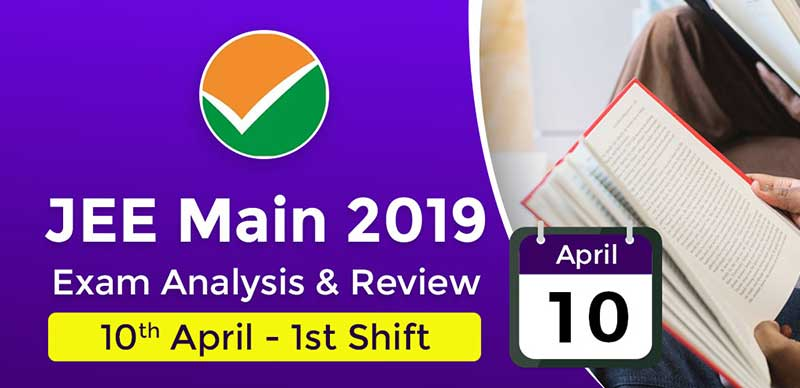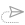### JEE Main 2019: Exam Analysis For Paper 1 (10th April - 1st Shift)

The 1st shift of JEE Main paper 1 has now ended for April 10, 2019. The analysis and reaction of students are provided here on this page.

Overall the paper in the 1st shift was from difficult to moderate level as per candidates.

Physics again was the toughest section whereas Mathematics was from difficult to moderate. However, chemistry yet again as the easiest of all.

The Exam analysis is important to estimate the performance in the exam.

JEE Main 2019 Paper 1: Exam Format

 Subject No of Questions Marks Mathematics 30 120 Chemistry 30 120 Physics 30 120 Total 90 360

Analysis for Physics:

The physics paper was the toughest of all three and had equal weightage from all section. More questions from Optics, while 2 questions from electrostatics.

One question was from logic gates and 2 Question from EM waves, ray optics. There also were questions from Speed and distance, Electric field and Series- parallel. 13 to 14 questions in physics were from class 12th.

A question was Mass and acceleration were given find out the Force. More of numerical based and graph related in physics.

Qs.1: What is the principle of Chromatography?

Analysis for chemistry

talking about chemistry it was the easiest section with equal weightage to organic and inorganic. More weightage was given to class 12th. Organic chemistry was very easy as reported by a student.

There were direct questions to write IUPAC names in chemistry. A question from Polymers that was direct and One or two tricky questions from reaction based.

Qs.1: Name the alloy which is used in making air craft?

Ans : Aluminium

Qs.2: What is the Electrophilic aromatic substitution reactivity order?

Qs.3: What is the reactivity order of SN1 reactions?

Analysis for Mathematics

A question in mathematics was repeated from Previous year exam. 4-5 Question from calculus in mathematics and Trigonometry was more in number.

There were 6 to 7 questions from differential, 3D geometry. Questions from Circle, integration, Relations and functions were there.

Qs.1: There were two points given on the graph P and Q on the intersection of two circles. Find the value of K

Qs.2: Range of x is given between (0,π), (0,π/2). Find the integral value of x in the given equation

Student Reaction:

• Overall, there were only 2-3 tricky questions in the exam.
• In Chemistry, direct questions were asked from Polymer
• For JEE Main 10 Apr 2019 Shift 1, many students are saying that Physics and Maths was difficult but Chemistry was easy
• In mathematics, there were some questions from Integration and Calculus
• Students said that organic was easy
• Number of questions from Trigonometry in the Mathematics section
• There was a question from Stratosphere.
• There was a question in which mass and acceleration was given and the students had to find the force.
• Students have commented that Physics was mostly numerical type
• In mathematics, there were no questions from Complex numbers, 2 questions from statistics and probability, 5 questions from Calculus.
• There was one question from Logic gate in the Physics section
• There was a question in which students had to give answer for the IUPAC name in the Chemistry section.
• In the Physics section, from mechanics, there were 3-4 questions, one question from the optic, there were no questions from semiconductor and 02 questions from electrostatics.
• In chemistry. students commented that there were major questions from Inorganic chemistry
• Integration and Limits section was a bit difficult
• There was equal weightage of Class 11th and 12th syllabi in Physics. However, the students felt that Class 12th syllabi covered major in physics.
• Chemistry was easy as usual.
• There were few questions repeated from the January attempt
• As per a student, physics was difficult17 September, 2021, 10:55 am##### JEE Advanced 2021: Supreme Court Gives Permission To Students to Appeal JEE Board for 3rd Attempt

16 September, 2021, 11:40 am##### JEE Advanced Objective Physics 2021 : Topic-Wise Physics Quick Revision Notes With MCQs, NATs & Their Solutions

16 September, 2021, 11:35 am##### JEE Advanced 2021 : Registration Begins For Foreign Nationals, Indians Must Wait Till JEE Main Result

15 September, 2021, 11:40 am##### JEE Main Session 4 Result 2021 Announced, 44 Students Scored 100 Percentile Marks

15 September, 2021, 11:06 am##### JEE Main 2021 : Result Expected To Be Declared Today Or Definitely Tomorrow, Confirms NTA

14 September, 2021, 11:00 amWe are providing something unique, useful and most importantly fun. By giving students a tool to find instant solutions to their doubts, we’re trying to make every student self-sufficient in practicing & completing their homework

### COMPANY×